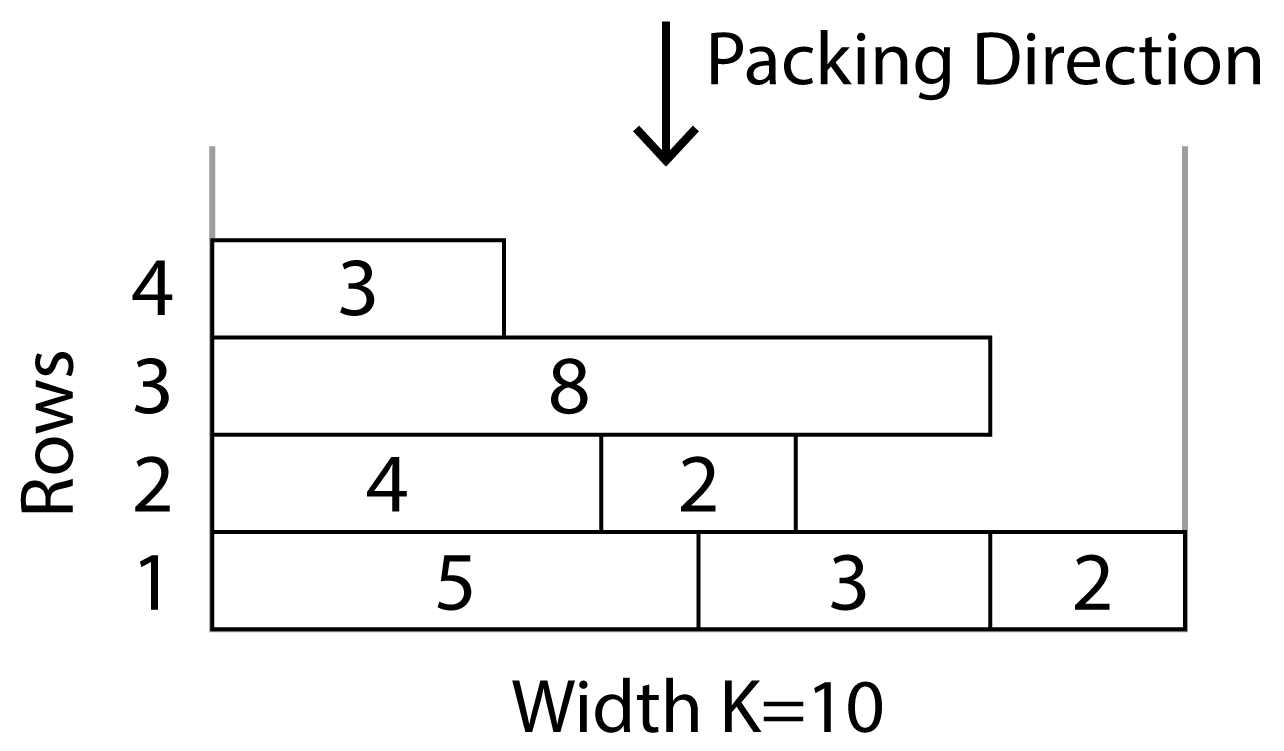Training Site# Packing Boxes

Input: Standard Input (stdin)
Output: Standard Output (stdout)
Memory limit: 256 megabytes
Time limit: 2.0 seconds

You are packing your car with boxes. You have $N$ boxes to pack. All boxes are one unit deep and the $i$-th box is $w_i$ units wide.

Your car is $K$ units wide and infinitely deep. You place each box into the car in order pushing them as far back, then as far to the left as possible. You do not rotate the boxes. Thus, the longest edge of a box always aligns with the width of the car.

If the next box you are packing fits (width-wise) through the gap you have created on the right then you will push the box through this gap as far back as you can, then as far to the left as you can. You can only push the next box past a row of packed boxes if there is a gap greater than or equal to the next box's width. If the next box does not fit in any gap then you will start a new row, and so on. Once a box is packed, you do not move it.

## Input

• The first line will contain two integers separated by a single space; these are $N$, the number of boxes to pack, and $K$, the width of the car.
• The next $N$ lines each contain a single integer $w_i$ (where $1 \le w_i \le K$), the width of the $i$-th box. These lines are in packing order.

## Output

On a single line, print the number of rows deep you require to pack all the boxes into your car using this technique.

• Subtask 1 (25%): $1 \le K \le 10$ and $1 \le N \le 20$.
• Subtask 2 (25%): $1 \le K \le 10$ and $1 \le N \le 200\,000$.
• Subtask 3 (50%): $1 \le K \le 100\,000$ and $1 \le N \le 250\,000$.

## Sample 1 Explanation

In the sample below, $N=7$ boxes require $4$ rows. The car width is $K=10$.

1. The 5 and 3 width boxes are placed on the first row and pushed left.
2. The width 4 box must go on a new row since $5 + 3 + 4 > 10$.
3. The width 8 box also goes on a new row.
4. The width 2 box can fit past row 3, so it slides down to complete the first row
5. Another width 2 box slides down to the second row and is pushed as far to the left $\leftarrow$ as possible.
6. The width 3 box cannot fit past row 3 (the gap is 2) so it makes a new row, requiring 4 rows in total.• ### Sample Input 1

7 10
5
3
4
8
2
2
3


### Sample Output 1

4

• ### Sample Input 2

4 5
4
2
1
2


### Sample Output 2

2